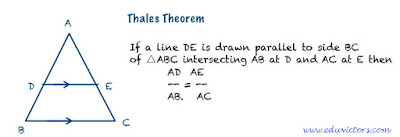## Triangles: Important Points You must Know1. Two figures are said to be similar if and only if they have same shape but not necessarily of same size.

2. All the congruent figures are similar but the converse is not true.

3. Two polygons of the same number of sides are similar if and only if
(i) their corresponding angles are equal
(ii) their corresponding sides are in proportion (same ratio).

4Basic Proportionality Theorem (Thales Theorem):
If a line is drawn parallel to one side of a triangle to intersect the other two sides in distinct points, then the other two sides are divided in the same ratio.(see figure above)

5. Converse of Basic Proportionality Theorem: If a line divides any two sides of a triangle in the same ratio, then the line is parallel to third side.

6. Similar Triangles: Two triangles are similar if and only if
i.  their corresponding angles are equal i.e. they are equi-angular and
ii. their corresponding sides are in the same ratio.

7. Criteria for establishing similarity of two triangles:
i.   AA (or AAA) criterion of similarity
ii.  SSS criterion
iii. SAS criterion
iv.  Altitude of right angle triangles

8. Similarity of triangles must be represented in correct correspondence of their vertices.

9. AA criterion of similarity: If two angles of a triangle are equal to two angles of another triangle, then the two triangles are similar.

10. SSS criterion of similarity: If the three sides of one triangle are proportional to the corresponding three sides of another triangle, then the two sides are similar.

11. SAS criterion of similarity: If one angle of a triangle is equal to the one angle of another triangle and the sides including those angles are proportional, then the two triangles are similar.

12. If a perpendicular is drawn from the vertex of the right angle of a triangle to the hypotenuse, then the triangles on each side of the altitude are similar to the whole triangle and to each other.

13. If two triangles are similar, then the ratio of their corresponding medians is equal to the ratio of their corresponding sides.

14. If two triangles are similar, then the ratio of their corresponding altitudes is equal to the ratio of their corresponding sides.

15. If two triangles are similar, then the ratio of their corresponding internal angle bisectors segments is equal to the ratio of their corresponding sides.

16. Areas of two similar triangles: The ratios of areas of two similar triangles is equal to the squares of the ratio of their corresponding sides.

17. If two triangles are similar, the the ratio of their areas:
i.   is equal to the ratio of the squares of their corresponding medians.
ii.  is equal to the ratio of the squares of their corresponding altitudes.
iii. is equal to the ratio of the squares of their corresponding internal angle bisector segments.

18. If the areas of two similar triangles are equal, then the triangles are congruent.

19Pythagoras Theorem: In a right angle, the square of the hypotenuse is equal to the sum of squares of the other two sides.

20. Converse of Pythagoras Theorem: In a triangle, if the square on one side is equal to the sum of the squares of other two sides, then the angle opposite to the first side is a right angle.

21. If a line segment drawn from the vertex of an angle of a triangle which divides the opposite sides in a ratio equal to the ratio of the sides containing the angle, then the line segment bisects the angle.